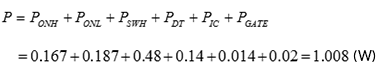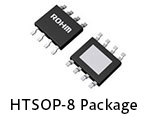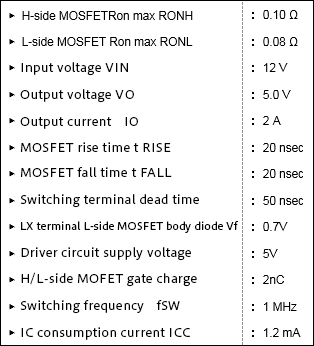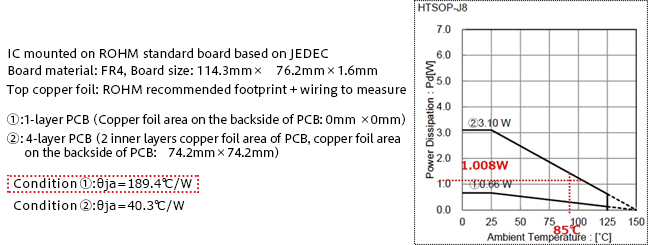# Heat Calculation for Package Selection: Example 1

2019.02.21

・Losses are determined for use in thermal design.

・Heat dissipation measures are taken in order that Tj does not exceed the absolute maximum rating.

Up to the preceding article, places where losses occur were discussed and methods for determining losses by calculation were explained. From this article, we will calculate the heat produced by losses thus determined, and will describe how to determine whether values are within maximum ratings for the conditions of actual use, and explain approaches to dealing with cases where maximum ratings are exceeded and the like. Originally, the reason for determining losses (efficiency) is to check whether the junction temperature Tj of an IC chip or transistor chip exceeds the maximum rating and confirm that a power supply circuit operates reliably and safely under the required conditions.

## Heat Calculation for Package Selection: Example 1

The results calculated in “Example of Power Loss Calculation for a Power Supply IC” are used. The conditions for which losses were calculated and the loss calculation results are indicated as well.

Total of power losses for the power supply ICAs in the table on the right, the output current IO is 2 A. The maximum ambient temperature of use is set to 85°C. Under these conditions, we consider the HTSOP-8 package for the power supply IC. The HTSOP-8 is a standard surface-mount SO package, with a metal plate exposed on the rear surface.Heat calculations ultimately determine the junction temperature Tj of the IC chip. Many IC data sheets provide graphs of allowable losses, but allowable losses also ultimately depend on Tj. Below is an equation for calculation of Tj. Of course this equation is not particularly special, but is merely a general equation representing Tj.

Tj＝Ta＋θja×P

Ta：Ambient temperature (℃), θja：Junction to ambient thermal resistance (℃/W), P：Power loss (W)

Thermal resistance information is needed for calculations. In many cases, IC data sheets provide this information together with conditions. Below is an extract from a data sheet. A graph of allowable losses under these conditions is also provided.We see that the thermal resistance θja differs with the number of layers of the mounting board. Here, a single-layer board is assumed, and so “condition ①” is used in calculations.

Tj＝Ta＋θja×P　⇒　85℃＋189.4℃/W×1.008W＝275.9℃

Tjmax is 150°C, and so the result is completely beyond what is acceptable. We tried inserting numerical values into the equation and calculating, but upon arranging the conditions, this result came up. The 1.008 W line that is added to the allowable loss graph already exceeds the allowable loss for condition ①. The position of intersection of the line for Ta=85°C and ① indicates the allowable power under condition ①, and it is clear at a glance that 1.008 W far exceeds this. Moreover, once it is understood that θja is 189.4°C/W, Tjmax rises above 150°C merely due to the heat generated for a loss of 1.008 W, and so from the calculations it is clear that in actuality, under these conditions, the device cannot be used.

However, the calculations are necessary in order to clarify what is possible, to what extent it is possible, and how to proceed to make it possible.

We have determined that, in order to complete the design while satisfying the required specifications, measures to address heat generation are necessary. In the next article, we will describe an example of such countermeasures.